# Exploratory Data Analysis in R Programming

Exploratory Data Analysis or EDA is a statistical approach or technique for analyzing data sets in order to summarize their important and main characteristics generally by using some visual aids. The EDA approach can be used to gather knowledge about the following aspects of data:

• Main characteristics or features of the data.
• The variables and their relationships.
• Finding out the important variables that can be used in our problem.

EDA is an iterative approach that includes:

• Generating questions about our data
• Searching for the answers by using visualization, transformation, and modeling of our data.
• Using the lessons that we learn in order to refine our set of questions or to generate a new set of questions.

### Implementation in R

In R Language, we are going to perform EDA under two broad classifications:

• Descriptive Statistics, which includes mean, median, mode, inter-quartile range, and so on.
• Graphical Methods, which includes histogram, density estimation, box plots, and so on.

Before we start working with EDA, we must perform the data inspection properly. Here in our analysis, we will be using the loafercreek from the soilDB package in R. We are going to inspect our data in order to find all the typos and blatant errors. Further EDA can be used to determine and identify the outliers and perform the required statistical analysis. For performing the EDA, we will have to install and load the following packages:

• “aqp” package
• “ggplot2” package
• “soilDB” package

We can install these packages from the R console using the install.packages() command and load them into our R Script by using the library() command. We will now see how to inspect our data and remove the typos and blatant errors.

#### Data Inspection for EDA in R

To ensure that we are dealing with the right information we need a clear view of your data at every stage of the transformation process. Data Inspection is the act of viewing data for verification and debugging purposes, before, during, or after a translation. Now let’s see how to inspect and remove the errors and typos from the data.

Example:

## R

 `# Data Inspection in EDA ` `# loading the required packages ` `library``(aqp) ` `library``(soilDB) ` ` `  `# Load from the the loakercreek dataset ` `data``(``"loafercreek"``)  ` ` `  `# Construct generalized horizon designations ` `n <- ``c``(``"A"``, ``"BAt"``, ``"Bt1"``, ``"Bt2"``, ``"Cr"``, ``"R"``) ` ` `  `# REGEX rules ` `p <- ``c``(``"A"``, ``"BA|AB"``, ``"Bt|Bw"``, ``"Bt3|Bt4|2B|C"``, ` `       ``"Cr"``, ``"R"``) ` ` `  `# Compute genhz labels and  ` `# add to loafercreek dataset ` `loafercreek\$genhz <- ``generalize.hz``( ` `                          ``loafercreek\$hzname,  ` `                          ``n, p) ` ` `  `# Extract the horizon table ` `h <- ``horizons``(loafercreek)  ` ` `  `# Examine the matching of pairing of  ` `# the genhz label to the hznames ` `table``(h\$genhz, h\$hzname) ` ` `  `vars <- ``c``(``"genhz"``, ``"clay"``, ``"total_frags_pct"``, ` `          ``"phfield"``, ``"effclass"``) ` `summary``(h[, vars]) ` ` `  `sort``(``unique``(h\$hzname)) ` `h\$hzname <- ``ifelse``(h\$hzname == ``"BT"``, ` `                   ``"Bt"``, h\$hzname)`

Output:

```> table(h\$genhz, h\$hzname)

2BCt 2Bt1 2Bt2 2Bt3 2Bt4 2Bt5 2CB 2CBt 2Cr 2Crt 2R  A A1 A2 AB ABt Ad Ap  B BA BAt BC BCt Bt Bt1 Bt2 Bt3 Bt4 Bw Bw1 Bw2 Bw3  C
A           0    0    0    0    0    0   0    0   0    0  0 97  7  7  0   0  1  1  0  0   0  0   0  0   0   0   0   0  0   0   0   0  0
BAt         0    0    0    0    0    0   0    0   0    0  0  0  0  0  1   0  0  0  0 31   8  0   0  0   0   0   0   0  0   0   0   0  0
Bt1         0    0    0    0    0    0   0    0   0    0  0  0  0  0  0   2  0  0  0  0   0  0   0  8  94  89   0   0 10   2   2   1  0
Bt2         1    2    7    8    6    1   1    1   0    0  0  0  0  0  0   0  0  0  0  0   0  5  16  0   0   0  47   8  0   0   0   0  6
Cr          0    0    0    0    0    0   0    0   4    2  0  0  0  0  0   0  0  0  0  0   0  0   0  0   0   0   0   0  0   0   0   0  0
R           0    0    0    0    0    0   0    0   0    0  5  0  0  0  0   0  0  0  0  0   0  0   0  0   0   0   0   0  0   0   0   0  0
not-used    0    0    0    0    0    0   0    0   0    0  0  0  0  0  0   0  0  0  1  0   0  0   0  0   0   0   0   0  0   0   0   0  0

CBt Cd Cr Cr/R Crt H1 Oi  R Rt
A          0  0  0    0   0  0  0  0  0
BAt        0  0  0    0   0  0  0  0  0
Bt1        0  0  0    0   0  0  0  0  0
Bt2        6  1  0    0   0  0  0  0  0
Cr         0  0 49    0  20  0  0  0  0
R          0  0  0    1   0  0  0 41  1
not-used   0  0  0    0   0  1 24  0  0

> summary(h[, vars])
genhz          clay       total_frags_pct    phfield            effclass
A       :113   Min.   :10.00   Min.   : 0.00   Min.   :4.90   very slight:  0
BAt     : 40   1st Qu.:18.00   1st Qu.: 0.00   1st Qu.:6.00   slight     :  0
Bt1     :208   Median :22.00   Median : 5.00   Median :6.30   strong     :  0
Bt2     :116   Mean   :23.67   Mean   :14.18   Mean   :6.18   violent    :  0
Cr      : 75   3rd Qu.:28.00   3rd Qu.:20.00   3rd Qu.:6.50   none       : 86
R       : 48   Max.   :60.00   Max.   :95.00   Max.   :7.00   NA's       :540
not-used: 26   NA's   :173                     NA's   :381

> sort(unique(h\$hzname))
 "2BCt" "2Bt1" "2Bt2" "2Bt3" "2Bt4" "2Bt5" "2CB"  "2CBt" "2Cr"  "2Crt" "2R"   "A"    "A1"   "A2"   "AB"   "ABt"  "Ad"   "Ap"   "B"
 "BA"   "BAt"  "BC"   "BCt"  "Bt"   "Bt1"  "Bt2"  "Bt3"  "Bt4"  "Bw"   "Bw1"  "Bw2"  "Bw3"  "C"    "CBt"  "Cd"   "Cr"   "Cr/R" "Crt"
 "H1"   "Oi"   "R"    "Rt"
```

Now proceed with the EDA.

#### Descriptive Statistics in EDA

For Descriptive Statistics in order to perform EDA in R, we will divide all the functions into the following categories:

• Measures of central tendency
• Measures of dispersion
• Correlation

We will try to determine the mid-point values using the functions under the Measures of Central tendency. Under this section, we will be calculating the mean, median, mode, and frequencies.

Example 1:

Now see the measures of central tendency in this example.

## R

 `# EDA ` `# Descriptive Statistics ` `# Measures of Central Tendency ` ` `  `#loading the required packages ` `library``(aqp) ` `library``(soilDB) ` ` `  `# Load from the the loakercreek dataset ` `data``(``"loafercreek"``)  ` ` `  `# Construct generalized horizon designations ` `n <- ``c``(``"A"``, ``"BAt"``, ``"Bt1"``, ``"Bt2"``, ``"Cr"``, ``"R"``) ` ` `  `# REGEX rules ` `p <- ``c``(``"A"``, ``"BA|AB"``, ``"Bt|Bw"``, ``"Bt3|Bt4|2B|C"``, ` `       ``"Cr"``, ``"R"``) ` ` `  `# Compute genhz labels and  ` `# add to loafercreek dataset ` `loafercreek\$genhz <- ``generalize.hz``( ` `                       ``loafercreek\$hzname,  ` `                       ``n, p) ` ` `  `# Extract the horizon table ` `h <- ``horizons``(loafercreek)  ` ` `  `# Examine the matching of pairing ` `# of the genhz label to the hznames ` `table``(h\$genhz, h\$hzname) ` ` `  `vars <- ``c``(``"genhz"``, ``"clay"``, ``"total_frags_pct"``,  ` `          ``"phfield"``, ``"effclass"``) ` `summary``(h[, vars]) ` ` `  `sort``(``unique``(h\$hzname)) ` `h\$hzname <- ``ifelse``(h\$hzname == ``"BT"``,  ` `                   ``"Bt"``, h\$hzname) ` ` `  `# first remove missing values  ` `# and create a new vector ` `clay <- ``na.exclude``(h\$clay) ` ` `  `mean``(clay) ` `median``(clay) ` `sort``(``table``(``round``(h\$clay)),  ` `     ``decreasing = ``TRUE``) ` `table``(h\$genhz) ` `# append the table with ` `# row and column sums ` `addmargins``(``table``(h\$genhz,  ` `                 ``h\$texcl)) ` ` `  `# calculate the proportions  ` `# relative to the rows, margin = 1  ` `# calculates for rows, margin = 2 calculates  ` `# for columns, margin = NULL calculates  ` `# for total observations ` `round``(``prop.table``(``table``(h\$genhz, h\$texture_class),  ` `                 ``margin = 1) * 100)  ` `knitr::``kable``(``addmargins``(``table``(h\$genhz, h\$texcl))) ` ` `  `aggregate``(clay ~ genhz, data = h, mean) ` `aggregate``(clay ~ genhz, data = h, median) ` `aggregate``(clay ~ genhz, data = h, summary)`

Output:

```> mean(clay)
 23.6713
> median(clay)
 22
> sort(table(round(h\$clay)), decreasing = TRUE)
25
41
> table(h\$genhz)

A      BAt      Bt1      Bt2       Cr        R not-used
113       40      208      116       75       48       26

cos   s  fs vfs lcos  ls lfs lvfs cosl  sl fsl vfsl   l sil  si scl  cl sicl  sc sic   c Sum
A          0   0   0   0    0   0   0    0    0   6   0    0  78  27   0   0   0    0   0   0   0 111
BAt        0   0   0   0    0   0   0    0    0   1   0    0  31   4   0   0   2    1   0   0   0  39
Bt1        0   0   0   0    0   0   0    0    0   1   0    0 125  20   0   4  46    5   0   1   2 204
Bt2        0   0   0   0    0   0   0    0    0   0   0    0  28   5   0   5  52    3   0   1  16 110
Cr         0   0   0   0    0   0   0    0    0   0   0    0   0   0   0   0   0    0   0   0   1   1
R          0   0   0   0    0   0   0    0    0   0   0    0   0   0   0   0   0    0   0   0   0   0
not-used   0   0   0   0    0   0   0    0    0   0   0    0   0   0   0   0   1    0   0   0   0   1
Sum        0   0   0   0    0   0   0    0    0   8   0    0 262  56   0   9 101    9   0   2  19 466

> round(prop.table(table(h\$genhz, h\$texture_class), margin = 1) * 100)

br   c  cb  cl  gr   l  pg scl sic sicl sil  sl spm
A          0   0   0   0   0  70   0   0   0    0  24   5   0
BAt        0   0   0   5   0  79   0   0   0    3  10   3   0
Bt1        0   1   0  23   0  61   0   2   0    2  10   0   0
Bt2        0  14   1  46   2  25   1   4   1    3   4   0   0
Cr        98   2   0   0   0   0   0   0   0    0   0   0   0
R        100   0   0   0   0   0   0   0   0    0   0   0   0
not-used   0   0   0   4   0   0   0   0   0    0   0   0  96

|         | cos|  s| fs| vfs| lcos| ls| lfs| lvfs| cosl| sl| fsl| vfsl|   l| sil| si| scl|  cl| sicl| sc| sic|  c| Sum|
|:--------|---:|--:|--:|---:|----:|--:|---:|----:|----:|--:|---:|----:|---:|---:|--:|---:|---:|----:|--:|---:|--:|---:|
|A        |   0|  0|  0|   0|    0|  0|   0|    0|    0|  6|   0|    0|  78|  27|  0|   0|   0|    0|  0|   0|  0| 111|
|BAt      |   0|  0|  0|   0|    0|  0|   0|    0|    0|  1|   0|    0|  31|   4|  0|   0|   2|    1|  0|   0|  0|  39|
|Bt1      |   0|  0|  0|   0|    0|  0|   0|    0|    0|  1|   0|    0| 125|  20|  0|   4|  46|    5|  0|   1|  2| 204|
|Bt2      |   0|  0|  0|   0|    0|  0|   0|    0|    0|  0|   0|    0|  28|   5|  0|   5|  52|    3|  0|   1| 16| 110|
|Cr       |   0|  0|  0|   0|    0|  0|   0|    0|    0|  0|   0|    0|   0|   0|  0|   0|   0|    0|  0|   0|  1|   1|
|R        |   0|  0|  0|   0|    0|  0|   0|    0|    0|  0|   0|    0|   0|   0|  0|   0|   0|    0|  0|   0|  0|   0|
|not-used |   0|  0|  0|   0|    0|  0|   0|    0|    0|  0|   0|    0|   0|   0|  0|   0|   1|    0|  0|   0|  0|   1|
|Sum      |   0|  0|  0|   0|    0|  0|   0|    0|    0|  8|   0|    0| 262|  56|  0|   9| 101|    9|  0|   2| 19| 466|
> aggregate(clay ~ genhz, data = h, mean)
genhz     clay
1     A 16.23113
2   BAt 19.53889
3   Bt1 24.14221
4   Bt2 31.35045
5    Cr 15.00000

> aggregate(clay ~ genhz, data = h, median)
genhz clay
1     A 16.0
2   BAt 19.5
3   Bt1 24.0
4   Bt2 30.0
5    Cr 15.0

> aggregate(clay ~ genhz, data = h, summary)
genhz clay.Min. clay.1st Qu. clay.Median clay.Mean clay.3rd Qu. clay.Max.
1     A  10.00000     14.00000    16.00000  16.23113     18.00000  25.00000
2   BAt  14.00000     17.00000    19.50000  19.53889     20.00000  28.00000
3   Bt1  12.00000     20.00000    24.00000  24.14221     28.00000  51.40000
4   Bt2  10.00000     26.00000    30.00000  31.35045     35.00000  60.00000
5    Cr  15.00000     15.00000    15.00000  15.00000     15.00000  15.00000
```

Now we will see the functions under Measures of Dispersion. In this category, we are going to determine the spread values around the mid-point. Here we are going to calculate the variance, standard deviation, range, inter-quartile range, coefficient of variance, and quartiles.

Example 2:

We shall see the measures of dispersion in this example.

## R

 `# EDA ` `# Descriptive Statistics ` `# Measures of Dispersion ` ` `  `# loading the packages ` `library``(aqp) ` `library``(soilDB) ` ` `  `# Load from the the loakercreek dataset ` `data``(``"loafercreek"``)  ` ` `  `# Construct generalized horizon designations ` `n <- ``c``(``"A"``, ``"BAt"``, ``"Bt1"``, ``"Bt2"``, ``"Cr"``, ``"R"``) ` ` `  `# REGEX rules ` `p <- ``c``(``"A"``, ``"BA|AB"``, ``"Bt|Bw"``, ``"Bt3|Bt4|2B|C"``, ` `       ``"Cr"``, ``"R"``) ` ` `  `# Compute genhz labels and add  ` `# to loafercreek dataset ` `loafercreek\$genhz <- ``generalize.hz``( ` `                     ``loafercreek\$hzname, ` `                       ``n, p) ` ` `  `# Extract the horizon table ` `h <- ``horizons``(loafercreek)  ` ` `  `# Examine the matching of pairing of  ` `# the genhz label to the hznames ` `table``(h\$genhz, h\$hzname) ` ` `  `vars <- ``c``(``"genhz"``, ``"clay"``, ``"total_frags_pct"``,  ` `          ``"phfield"``, ``"effclass"``) ` `summary``(h[, vars]) ` ` `  `sort``(``unique``(h\$hzname)) ` `h\$hzname <- ``ifelse``(h\$hzname == ``"BT"``,  ` `                   ``"Bt"``, h\$hzname) ` ` `  `# first remove missing values  ` `# and create a new vector ` `clay <- ``na.exclude``(h\$clay) ` `var``(h\$clay, na.rm=``TRUE``) ` `sd``(h\$clay, na.rm = ``TRUE``) ` `cv <- ``sd``(clay) / ``mean``(clay) * 100 ` `cv ` `quantile``(clay) ` `range``(clay) ` `IQR``(clay)`

Output:

```> var(h\$clay, na.rm=TRUE)
 64.89187

> sd(h\$clay, na.rm = TRUE)
 8.055549

> cv
 34.03087

> quantile(clay)
0%  25%  50%  75% 100%
10   18   22   28   60

> range(clay)
 10 60

> IQR(clay)
 10
```

Now we will work on Correlation. In this part, all the calculated correlation coefficient values of all variables in tabulated as the Correlation Matrix. This gives us a quantitative measure in order to guide our decision-making process.

Example 3:

We shall now see the correlation in this example.

## R

 `# EDA ` `# Decriptive Statistics ` `# Correlation ` ` `  `# loading the required packages ` `library``(aqp) ` `library``(soilDB) ` ` `  `# Load from the the loakercreek dataset ` `data``(``"loafercreek"``)  ` ` `  `# Construct generalized horizon designations ` `n <- ``c``(``"A"``, ``"BAt"``, ``"Bt1"``, ``"Bt2"``, ``"Cr"``, ``"R"``) ` ` `  `# REGEX rules ` `p <- ``c``(``"A"``, ``"BA|AB"``, ``"Bt|Bw"``, ``"Bt3|Bt4|2B|C"``, ` `       ``"Cr"``, ``"R"``) ` ` `  `# Compute genhz labels and add  ` `# to loafercreek dataset ` `loafercreek\$genhz <- ``generalize.hz``( ` `                       ``loafercreek\$hzname,  ` `                     ``n, p) ` ` `  `# Extract the horizon table ` `h <- ``horizons``(loafercreek)  ` ` `  `# Examine the matching of pairing  ` `# of the genhz label to the hznames ` `table``(h\$genhz, h\$hzname) ` ` `  `vars <- ``c``(``"genhz"``, ``"clay"``, ``"total_frags_pct"``, ` `          ``"phfield"``, ``"effclass"``) ` `summary``(h[, vars]) ` ` `  `sort``(``unique``(h\$hzname)) ` `h\$hzname <- ``ifelse``(h\$hzname == ``"BT"``,  ` `                   ``"Bt"``, h\$hzname) ` ` `  `# first remove missing values  ` `# and create a new vector ` `clay <- ``na.exclude``(h\$clay) ` ` `  `# Compute the middle horizon depth ` `h\$hzdepm <- (h\$hzdepb + h\$hzdept) / 2  ` `vars <- ``c``(``"hzdepm"``, ``"clay"``, ``"sand"``,  ` `          ``"total_frags_pct"``, ``"phfield"``) ` `round``(``cor``(h[, vars], use = ``"complete.obs"``), 2)`

Output:

```                hzdepm  clay  sand total_frags_pct phfield
hzdepm            1.00  0.59 -0.08            0.50   -0.03
clay              0.59  1.00 -0.17            0.28    0.13
sand             -0.08 -0.17  1.00           -0.05    0.12
total_frags_pct   0.50  0.28 -0.05            1.00   -0.16
phfield          -0.03  0.13  0.12           -0.16    1.00
```

Hence, the above three classifications deal with the Descriptive statistics part of EDA. Now we shall move on to the Graphical Method of representing EDA.

#### Graphical Method in EDA

Since we have already checked our data for missing values, blatant errors, and typos, we can now examine our data graphically in order to perform EDA. We will see the graphical representation under the following categories:

• Distributions
• Scatter and Line plot

Under the Distribution, we shall examine our data using the bar plot, Histogram, Density curve, box plots, and QQplot.

Example 1:

We shall see how distribution graphs can be used to examine data in EDA in this example.

## R

 `# EDA Graphical Method Distributions ` ` `  `# loading the required packages ` `library``(``"ggplot2"``) ` `library``(aqp) ` `library``(soilDB) ` ` `  `# Load from the the loakercreek dataset ` `data``(``"loafercreek"``)  ` ` `  `# Construct generalized horizon designations ` `n <- ``c``(``"A"``, ``"BAt"``, ``"Bt1"``, ``"Bt2"``, ``"Cr"``, ``"R"``) ` ` `  `# REGEX rules ` `p <- ``c``(``"A"``, ``"BA|AB"``, ``"Bt|Bw"``, ``"Bt3|Bt4|2B|C"``, ` `       ``"Cr"``, ``"R"``) ` ` `  `# Compute genhz labels and add  ` `# to loafercreek dataset ` `loafercreek\$genhz <- ``generalize.hz``( ` `                       ``loafercreek\$hzname, n, p) ` ` `  `# Extract the horizon table ` `h <- ``horizons``(loafercreek)  ` ` `  `# Examine the matching of pairing  ` `# of the genhz label to the hznames ` `table``(h\$genhz, h\$hzname) ` ` `  `vars <- ``c``(``"genhz"``, ``"clay"``, ``"total_frags_pct"``,  ` `          ``"phfield"``, ``"effclass"``) ` `summary``(h[, vars]) ` ` `  `sort``(``unique``(h\$hzname)) ` `h\$hzname <- ``ifelse``(h\$hzname == ``"BT"``,  ` `                   ``"Bt"``, h\$hzname) ` ` `  `# graphs ` `# bar plot ` `ggplot``(h, ``aes``(x = texcl)) +``geom_bar``() ` ` `  `# histogram ` `ggplot``(h, ``aes``(x = clay)) + ` `  ``geom_histogram``(bins = ``nclass.Sturges``(h\$clay)) ` ` `  `# density curve ` `ggplot``(h, ``aes``(x = clay)) + ``geom_density``() ` ` `  `# box plot ` `ggplot``(h, (``aes``(x = genhz, y = clay))) + ` `geom_boxplot``() ` ` `  `# QQ Plot for Clay ` `ggplot``(h, ``aes``(sample = clay)) +  ` `geom_qq``() + ` `geom_qq_line``()`

Output: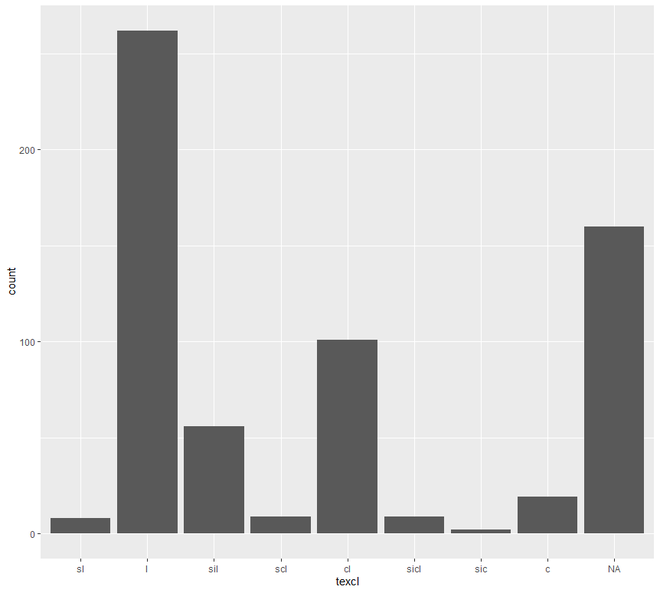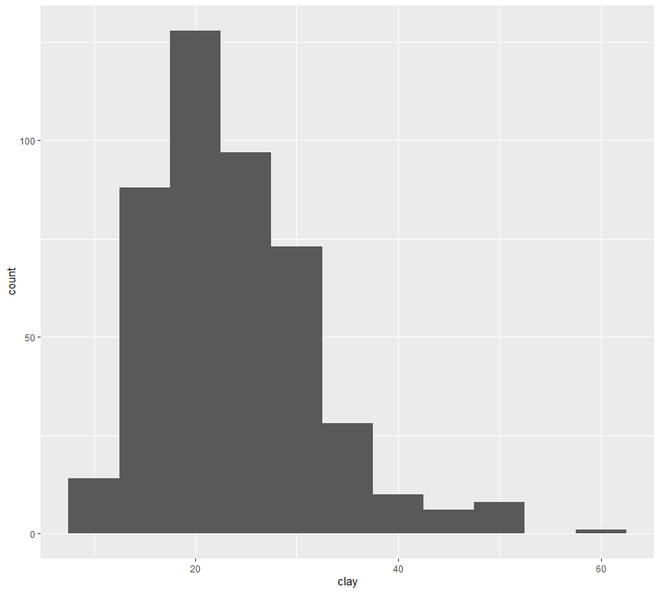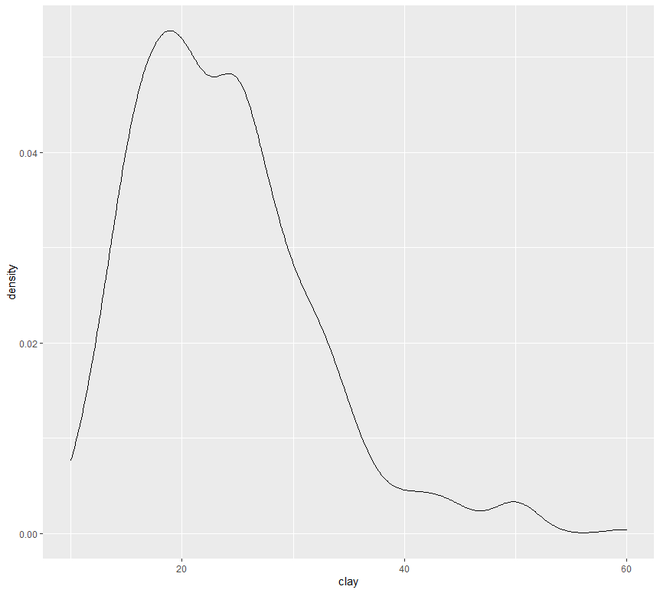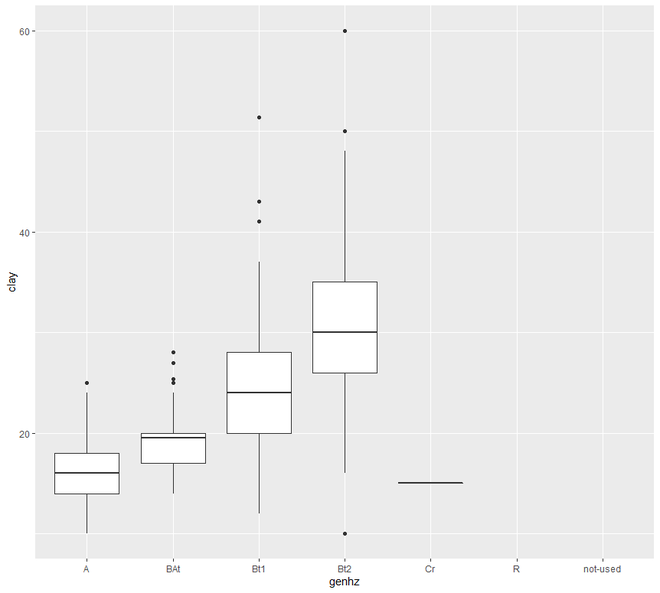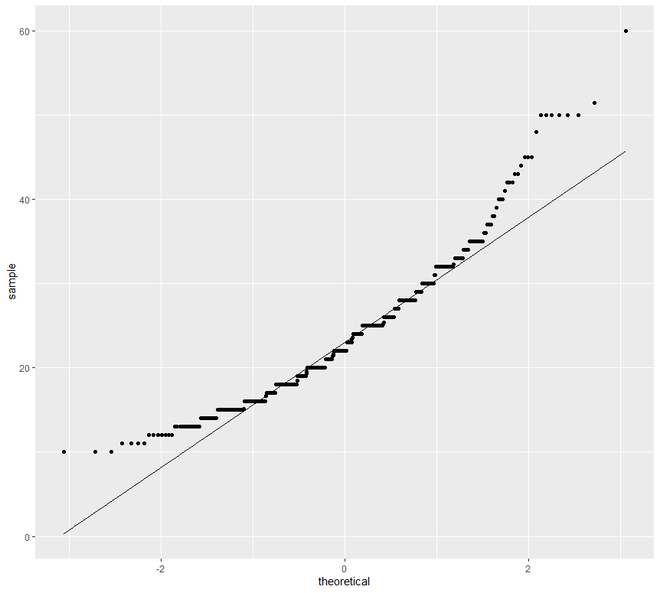Now we will move on to the Scatter and Line plot. In this category, we are going to see two types of plotting,- scatter plot and line plot. Plotting points of one interval or ratio variable against variable are known as a scatter plot.

Example 2:

We shall now see how to use scatter and line plots to examine our data.

## R

 `# EDA ` `# Graphical Method ` `# Scatter and Line plot ` ` `  `# loading the required packages ` `library``(``"ggplot2"``) ` `library``(aqp) ` `library``(soilDB) ` ` `  `# Load from the the loakercreek dataset ` `data``(``"loafercreek"``)  ` ` `  `# Construct generalized horizon designations ` `n <- ``c``(``"A"``, ``"BAt"``, ``"Bt1"``, ``"Bt2"``, ``"Cr"``, ``"R"``) ` ` `  `# REGEX rules ` `p <- ``c``(``"A"``, ``"BA|AB"``, ``"Bt|Bw"``, ``"Bt3|Bt4|2B|C"``, ` `       ``"Cr"``, ``"R"``) ` ` `  `# Compute genhz labels and add  ` `# to loafercreek dataset ` `loafercreek\$genhz <- ``generalize.hz``( ` `                       ``loafercreek\$hzname, n, p) ` ` `  `# Extract the horizon table ` `h <- ``horizons``(loafercreek)  ` ` `  `# Examine the matching of pairing  ` `# of the genhz label to the hznames ` `table``(h\$genhz, h\$hzname) ` ` `  `vars <- ``c``(``"genhz"``, ``"clay"``, ``"total_frags_pct"``, ` `          ``"phfield"``, ``"effclass"``) ` `summary``(h[, vars]) ` ` `  `sort``(``unique``(h\$hzname)) ` `h\$hzname <- ``ifelse``(h\$hzname == ``"BT"``, ` `                   ``"Bt"``, h\$hzname) ` ` `  `# graph ` `# scatter plot ` `ggplot``(h, ``aes``(x = clay, y = hzdepm)) + ` `  ``geom_point``() + ` `  ``ylim``(100, 0) ` ` `  `# line plot ` `ggplot``(h, ``aes``(y = clay, x = hzdepm,  ` `                     ``group = peiid)) + ` `geom_line``() + ``coord_flip``() + ``xlim``(100, 0)`

Output: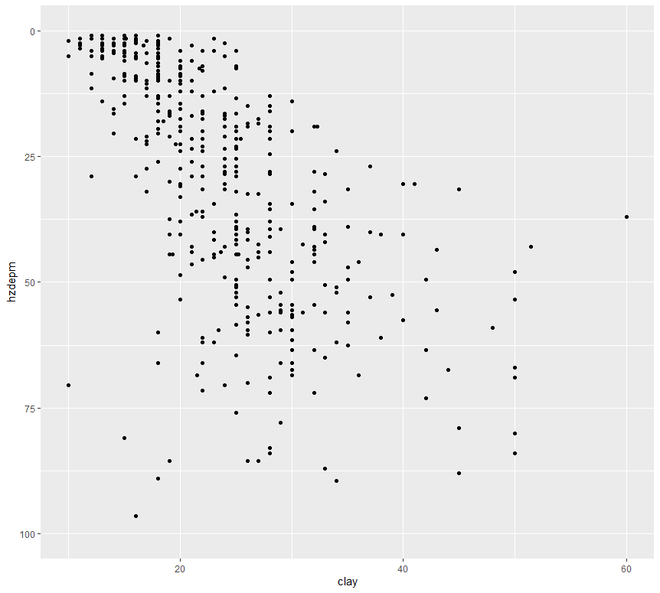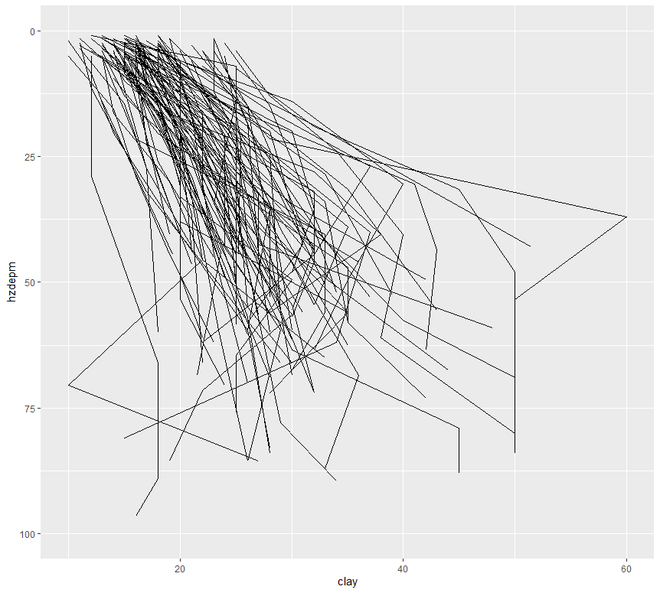My Personal Notes arrow_drop_upCheck out this Author's contributed articles.

If you like GeeksforGeeks and would like to contribute, you can also write an article using contribute.geeksforgeeks.org or mail your article to contribute@geeksforgeeks.org. See your article appearing on the GeeksforGeeks main page and help other Geeks.

Please Improve this article if you find anything incorrect by clicking on the "Improve Article" button below.

Article Tags :
Practice Tags :

Be the First to upvote.

Please write to us at contribute@geeksforgeeks.org to report any issue with the above content.